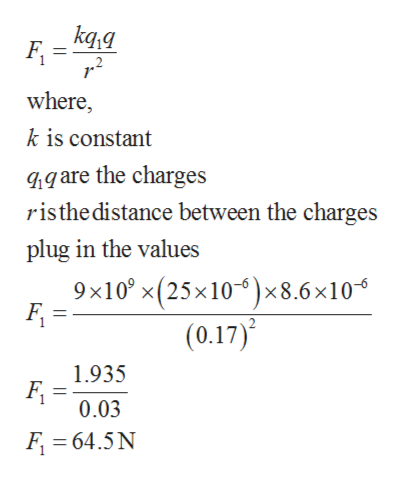# Two point charges are fixed on the y axis: a negative point charge q1 = -25 μC at y1 = +0.17 m and a positive point charge q2 at y2 = +0.33 m. A third point charge q = +8.6 μC is fixed at the origin. The net electrostatic force exerted on the charge q by the other two charges has a magnitude of 25 N and points in the +y direction. Determine the magnitude of q2.

Question
108 views

Two point charges are fixed on the y axis: a negative point charge q1 = -25 μC at y1 = +0.17 m and a positive point charge q2 at y2 = +0.33 m. A third point charge q = +8.6 μC is fixed at the origin. The net electrostatic force exerted on the charge q by the other two charges has a magnitude of 25 N and points in the +y direction. Determine the magnitude of q2.

check_circle

star
star
star
star
star
1 Rating
Step 1

Given:

Charge on q1 = -25 µC

Charge on q = 8.6 µC

Position of charge q1, y1 = 0.17 m

Position of charge q2, Y2 = 0.33 m

Electrostatic force on q, F = 25 N

Step 2

q1 exerts a force F1 on q towards +y direction and can be calculated ashelp_outlineImage Transcriptionclosekq,q where, k is constant gare the charges risthe distance between the charges plug in the values 9x10 x(25x10)x 8.6 x10 (0.17) 1.935 F 0.03 F 64.5N fullscreen
Step 3

Total force on q is F and Force due to q1 is F1. Therefore, force due t...

### Want to see the full answer?

See Solution

#### Want to see this answer and more?

Solutions are written by subject experts who are available 24/7. Questions are typically answered within 1 hour.*

See Solution
*Response times may vary by subject and question.
Tagged in
SciencePhysics

### Electric Charges and Fields